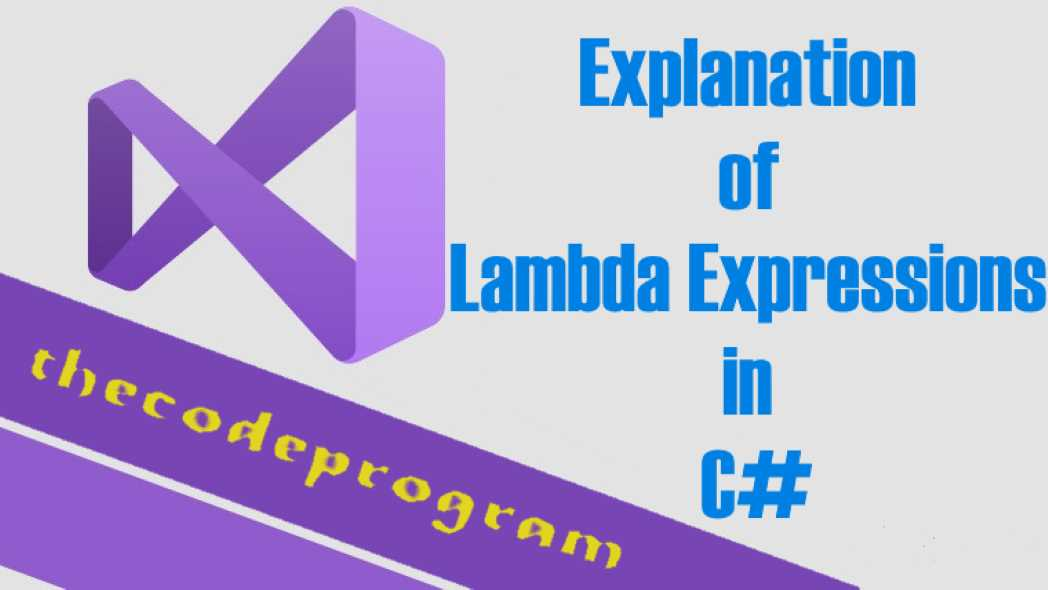## Explanation of Lambda Expressions in C#

Hello everyone, in this article we are going to talk about Lampda Expressions in C#. We will talk what Lambda is and what can we do with Lampda Expressions with an example in C#.

Let's get started.

Lambda expressions are similar with anonymous methods in C#. You can use it without declaring any variable type. You do not need to specify the type. This lets you to increase the flexibility of the code. In C# language normally equasitions right side is input and left side is expression, but in Lambdas, left side is input and right side is expression.

We can define the lambda expressions and lambda statements. In expressions we will make the operations directly. In lambda statement we will use it as if-else if block.

Let's write some code below.

In this example we are going to make a simple Odd-Even Number application with Lambda.

First we declare the list and constructor method and we are going to make callbacks from the constructor. Below example you will see it.

``````
List<int> numbers;

public OddEven_Numbers(List<int> _numbers)
{
this.numbers = _numbers;
list_numbers();
expression_operation();
statement_operation();
}

private void list_numbers()
{
//First see what the numbers are.
Console.WriteLine("Lambda Numbers : ");
for (int i = 0; i < this.numbers.Count; i++)
{
Console.WriteLine(this.numbers[i] + "");
}
}
``````

Here we see the expressions of the lambda. Here we are going to make a mathematical calculations for all items without any statements. So we call it as lambda expressions. We will multiply all numbers int eh list by two. In here we used foreach but if you want you can use the for loop or another loop to write all of the values.

``````
private void expression_operation()
{
Console.WriteLine(Environment.NewLine + "Lambda Expressions  ");
//Here we see the expressions of the lambda
//Here we are going to make a mathematical calculations for all items without any statements
//So we call it as lambda expressions
//We will multiply all numbers int eh list by two
//In here we used foreach but if you want you can use the for loop or another loop to write all of the values.
var multiply_by_2 = numbers.Select(x => x * 2);
foreach (var item in multiply_by_2)
{
Console.WriteLine(item);
}
}
``````

Here we see the lambda statements. We are going to writeline the numbers are accomodate the statement which we specified in lambda. So we call it as lambda statements

``````
private void statement_operation()
{
Console.WriteLine(Environment.NewLine + "Lambda Statements  ");
//Here we see the lambda statements.
//We are going to writeline the numbers are accomodate the statement which we specified in lambda
//So we call it as lambda statements
List<int> odd_number = numbers.FindAll(x => (x % 2) == 1);
foreach (var item in odd_number)
{
Console.WriteLine(item);
}
}
``````

You can also use lambda with user defined classes. Below you can see the example class. I defined an aircraft class in this example and ı will list all of them firstly, then ı will list the aircrafts which are in service.

Below you can see the example class.

``````
using System;
using System.Collections.Generic;

namespace Lambda_Example
{
class Aircraft
{
public string Brand;
public string MajorModel;
public string MinorModel;
public bool isInService;

public Aircraft(string _brand, string _major, string _minor, bool _isInService)
{
Brand = _brand;
MajorModel = _major;
MinorModel = _minor;
isInService = _isInService;
}
}

class UserDefined
{
public List<Aircraft> aircrafts;

public UserDefined()
{
aircrafts = new List<Aircraft>()
{
new Aircraft("Airbus", "A330", "300", true),
new Aircraft("Boeing", "777", "300ER", true),
new Aircraft("Boeing", "707", "10", false)
};
listAllAircrafts();
listAircraftsInService();
}

private void listAllAircrafts()
{
//Here first we print all aircrafts which are inside the list.
Console.WriteLine(Environment.NewLine + "All Aircraft List : ");
for (int i = 0; i < this.aircrafts.Count; i++)
{
Console.WriteLine(this.aircrafts[i].Brand + " " +
this.aircrafts[i].MajorModel + " " +
this.aircrafts[i].MinorModel + " " +
this.aircrafts[i].isInService);
}
}

private void listAircraftsInService()
{
//Here are going to make a statement to specify the in service aircrafts.
//We use here the class which defined by the user.
Console.WriteLine(Environment.NewLine + "All aircrafts which are in service : ");
List<Aircraft> aircraftInService = aircrafts.FindAll(x => (x.isInService) == true);
foreach (var item in aircraftInService)
{
Console.WriteLine(item.Brand + " " +
item.MajorModel + " " +
item.MinorModel + " " +
item.isInService);
}
}
}
}
``````
In the main program we will call the both classes with below code block.
``````
using System;
using System.Collections.Generic;

namespace Lambda_Example
{
class Program
{
static void Main(string[] args)
{
List<int> lstNumbers = new List<int>() { 1, 2, 7, 11, 24, 77, 33, 93 };
OddEven_Numbers _oddEvenNumbers = new OddEven_Numbers(lstNumbers);

Console.WriteLine("------------------------------------------");

UserDefined userDefined = new UserDefined();

}
}
}
``````

You can reach the example application on Github : https://github.com/thecodeprogram/LambdaExample_CSharp

Have a good healthy days.

Burak Hamdi TUFAN.

04/08/2020

17/05/2021

12/06/2023